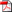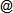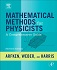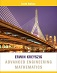ENGR 520School of Engineering
CUA, Fall 2013
Course Information - Description - Textbooks - Topics
Organization - Grading - Schedule, Notes & Assignments - ABET Criteria
Download SyllabusCourse Information•  Credits: 3.00 •  Schedule: Thursday evenings, 6:40–9:10pm •  Classroom: Gowan 400 •  Number: 01-LEC (1852) •  Capacity: 25 •  Instructor: Dr. René Doursat •  Email: doursatcua.edu •  Phone: (202) 319-5160 •  Office: for now: Pangborn 208-C (later, probably next week: Pangborn 121) •  Hours: Wednesday & Friday, 11:00am–12:30pm •  T. A.: Amie Lin •  Email: 13lincardinalmail.cua.edu

Description & Objectives

The objective of this course is to provide an introduction to the mathematical methods that will be needed in subsequent graduate-level courses in engineering. Emphasis is placed on understanding the concepts for solving first- and second-order differential equations (ODEs), as opposed to extricating an answer from math packages such as Mathematica or MATLAB. The philosophy of understanding the underlying concepts of the math is emphasized throughout the course and is extended to the concept of integration in the complex plane and the origination of the Fourier/Laplace transforms as well as their inverse transforms. Additionally, various special functions are examined including: Gamma, Beta, Bessel, and Legendre (and associated) polynomials. The process of separation of variables in partial differential equations (PDEs) is covered in the three major coordinate systems with application to the Laplace, heat, and wave equations.

Textbooks

• Required TextbookArfken, G. B., Weber, H. J. & Harris, F. E. (2012) Mathematical Methods for Physicists (7th Edition): A Comprehensive Guide. Academic Press (ISBN: 978-0123846549).
• Optional TextbookKreyszig, E. (2011) Advanced Engineering Mathematics (10th Edition). Wiley (ISBN: 978-0470458365).

Topics Covered (Tentative)

• First-order ODEs
• Second-order ODEs, Wronskian
• Power series solutions, Bessel functions, Legendre polynomials
• Convergence, regular and essential singularities
• Method of Frobenius, associated Legendre equation
• Integration techniques, complex Z- and W-planes, multi-valued functions and branch cuts
• Cauchy-Riemann equations, deformation of contours, integration about a singularity
• Taylor and Laurent expansions of f(z), Jordan's lemma, Gamma and Beta functions
• Fourier series solutions for periodic functions, Fourier and inverse Fourier transforms
• Laplace and inverse Laplace transforms
• Classification of PDEs
• Separation of variables in Cartesian, cylindrical, and spherical coordinates
• Solution of model equations: Laplace's, wave and heat equations
• Extended discussion of Bessel functions to include development of second solutions, asymptotic representations, Hankel functions (cylindrical and spherical)

Organization
• The use of laptops, tablets, cell phones, cameras, recorders, or other devices is not allowed during any of the meetings (lectures and exams). Bring paper and pens!

• Homework will be assigned at the end of every class (i.e. weekly, except during recess) and due at the beginning of the next class. It will consist of exercises and practice questions intended to test and affirm your knowledge of the course content. Collaboration on homework is accepted but must be acknowledged (cite your study partners), and you must write your solutions individually. Your paper must be written neatly, organized, and stapled together. Late homework will be penalized (10% per day).

• Exams will include one midterm during the semester (see Schedule for tentative date) and one final. Make-up exams will not be given except in cases of documented emergency.

• Important dates:
• Last day to add/drop courses → Sep 6
• Midterm Exam → Oct 10
• Last day to withdraw → Nov 8
• Final Exam → Dec 12, 5:45-7:45pm

Both grading policy and scale are subject to change. Failure in either the practice component (homework) or the lecture component (quizzes and exams) will result in an F for the whole course.

 Homework 40% Midterm Exam 25% Final Exam 35%

 100 → 90 A, A- 89 → 80 B+, B, B- 79 → 70 C 69 → 0 F

Schedule, Notes & Assignments (Tentative)

This is a tentative schedule, subject to readjustment depending on the time we actually spend in class covering the topics. Please check again regularly. Course notes (if any), assignments and exam solutions are posted on the CUA Blackboard, under "Math Analysis.../Course Documents" and ".../Assignments". Permanent reading assignment: it is assumed that you are familiar with the contents of the course notes of all past meetings.

 Week Date Topics & Course Notes (moved to the CUA Blackboard, under "Math Analysis.../Course Documents") Reading Assignments Homework & Exams (in Blackboard, ".../Assignments") 1Thu, Aug 29 0. Preliminaries: the binomial theorem, derivatives of the $\ln$ function, Euler's theorem, the imaginary $i\!$, operations in complex space, conversion between Cartesian form $a+ib$ and polar form $re^{i\theta}$. Definition of linear and nonlinear differential equations. 1. First-order differential equations: Special case of separable variables. Solving the general linear case with an integrating factor $\alpha(x)$.• read Ch. 7, pp. 329-339• HW1 • HW1 key 2 Thu, Sep 5 1. First-order differential equations: (cont'd) case of exact equations, inexact equations using an integrating factor $\mu(x)$ to restore exactness, "pseudo-linear" types $y'=f(ax+by+c)$, Bernoulli equations, "homogeneous-degree" functions $P$ and $Q$ and balanced equations. Discussion of explicit/implicit equations versus explicit/implicit solutions. • read First-Order ODEs• read Paul's Notes: Intro • read Paul's Notes: 1st-Order   (first 5 sections) • HW2 • HW2 key 3 Thu, Sep 12 2. Second-order differential equations: Homogeneous linear equations with constant coefficients and characteristic polynomial. General homogeneous linear equations with one known solution and reduction of order to find another solution. Inhomogeneous linear equations: $y$ missing and reduction to first-order linear. General inhomogeneous case: solving homogeneous version first, then finding a particular solution by undetermined coefficients. • read Second-Order ODEs• read Ch. 7, pp. 342-345 • read Ch. 7, pp. 375-378 • read Paul's Notes: 2nd-Order   (except "Fundam.", "Wronskian",    "Variation" and "Mechanical") • HW3 • HW3 key 4 Thu, Sep 19 1.-2. Reviewing and completing DE methods: First-order: restoring exactness with an integrating factor $\mu(y)$ or $x^n y^m$. Second-order: solving the linear inhomogeneous case: finding a particular solution by variation of parameters. Using the Wronskian to demonstrate linear independence and determine an unknown solution from a known one. General case with $x$ missing. 3. Power series solutions: Discussion of convergence and the radius of convergence. Power series solutions of first-order DEs. • read Ch. 7, pp. 358-363 • read Paul's Notes: 2nd-Order   ("Fundamental", "Wronskian",    "Variation" and "Mechanical") • HW4 • HW4 key 5 Thu, Sep 26 The Wave/Helmholtz equation: general solutions by separation of variables. Cartesian coordinates: obtaining planar waves. Cylindrical coordinates: obtaining Bessel's differential equations. Spherical coordinates: obtaining Legendre's equations. 3. Power series solutions: (cont'd) Second-order DEs: Development of Bessel function of order 0, $J_0(x)$. Higher-order Bessel functions the first type $J_\nu(x)$. Finding Bessel functions of the second type (Neumann functions) $N_\nu(x)$ by reduction of order. • read Ch. 9, pp. 414-426 • read Riffe's Notes: Wave Eq.   (Lectures 18-22) • read Paul's Notes: Series   (except "Euler Equations") • HW5 • HW5 key 6 Thu, Oct 3 3. Power series solutions: (cont'd) Convergence issues for the power series solution of Legendre at $\pm&space;1$. Resolution of convergence using truncated (polynomial) solutions for integer parameter values. Non-essential vs. essential singularities. Frobenius method. Associated Legendre equation. Solution about the weak singular points. Complete (convergent) solutions using associated Legendre polynomials. 4. Other integration techniques: integrating $\exp(-ax^2)$, $\exp(-x^\alpha)$, $\exp(-x)/x$, introduction of a convergence factor (as in the Laplace transform). • read Ch. 1, pp. 65-74 • MT Review 7 Thu, Oct 10 Midterm Exam (2h) 8 Thu, Oct 17 5. Analytic functions in the complex plane: Cartesian and polar form of complex numbers, DeMoivre's formula, discussion of the $z$-plane and the $w$-plane. Multi-valued functions and the need for branch cuts to maintain single-valuedness. Definition of analytic functions. The Cauchy-Riemann equations. Cauchy's integral theorem. Deforming contours. Integration about a singular point. Poles of higher orders. Numerous worked examples explaining these concepts. The "unit circle" method. 9 Thu, Oct 24 Taylor series expansion of $f(z)$. Laurent expansion of $f(z)$. Jordan's lemma. More worked examples. The Gamma function $\Gamma$: relationship to factor notation, integrals solvable using $\Gamma$ functions. The Beta function $\operatorname{B}$ and solution of related integrals. 10 Thu, Oct 31 Fourier series solution of periodic functions. The Fourier series in complex exponential form. Worked examples. Development of the Fourier transform using the complex exponential form of the Fourier series. Definition of the Fourier Transform and the inverse Fourier Transform. Use of the complex plane for finding the inverse transform. 2D and 3D Fourier transforms. Properties of Fourier transforms: shifting, differentiation, integration. 11 Thu, Nov 7 Introduction of the delta function $\delta$ and its use in determining the Fourier transform of $\sin$ and $\cos$ functions. The Laplace transform and its need for certain unbounded functions. Definition of the inverse Laplace transform. Finding the inverse Laplace transform by using the Bromwich integral. Application examples. 12 Thu, Nov 14 Diffusion/Heat and Laplace equations: Separation of variables in Cartesian coordinates. Separation of variables in cylindrical coordinates. Separation of variables in spherical coordinates. Power series solutions. 13 Thu, Nov 21 More discussion of Bessel functions: negative orders, fractional orders, linear independence of fractional orders. Definition of the second homogenous solution when integer orders are used. Asymptotic representations of Bessel functions. The need for Hankel functions (cylindrical coordinates) and spherical Hankel functions (spherical coordinates). Worked examples, particularly in cylindrical coordinates. 14 Thu, Nov 28 Thanksgiving recess - no class 15 Thu, Dec 5 Review 16 Thu, Dec 125:45-7:45pm Final Exam (2h)

ABET Criteria

• Contributions of course to meeting the professional components: This graduate level engineering mathematics class develops the core competency of mathematics needed for a graduate-level study of electrical, mechanical, civil, chemical, computer, or biomedical engineering in subsequent applied courses. This class contains a thorough review of solutions for differential equations. Integration into the complex plane is introduced and developed with connections made to inverse Fourier and Laplace transforms. Fourier and Laplace transforms are developed from first principles so that the underpinnings of the theory are clear and students have a deeper understanding of the concepts and are not simply solving equations using tables of transforms. Separation of variables in the three major coordinate systems is developed from first concepts and applied to practical engineering equations such as the Laplace, wave, and diffusion equations.

• Relationship of the course to program objectives: This course attempts to meet the stated departmental objectives:

1. An appreciation of the mathematical tools needed for graduate engineering studies
2. A working knowledge of advanced mathematical techniques

• Expected learning outcomes: Upon completion of the course, students should demonstrate the ability to:

1. CO-1: Solve first- and second-order differential equations
2. CO-2: Solve differential equations using power series
3. CO-3: Work with integration techniques using the complex plane
4. CO-4: Work with the Gamma and Beta functions
5. CO-5: Develop Fourier and Laplace transforms and inverse transforms
6. CO-6: Work with separation of variables applied to solving differential equations

• Course outcome/ABET outcome matrix: The Matrix below shows how this course contributes covers the 11 ABET Outcomes:

 ABET  01 ABET  02 ABET  03 ABET  04 ABET  05 ABET  06 ABET  07 ABET  08 ABET  09 ABET  10 ABET  11 CO-1 X X X X X X CO-2 X X X X X X CO-3 X X X X X X CO-4 X X X X X X CO-5 X X X X X X CO-6 X X X X X X

• Outcome assessment: The course employs the following mechanisms to assess the above learning outcomes:

1. Homework is assigned and graded weekly to assess the level of student understanding of topics covered during the week. The learning outcomes are also exhibited through the results of the several exams given during the semester and the final examination.
2. The instructor frequently asks students if they understand the lectures.
3. The overall assessment of the course is done through the university's Student Course Evaluation process.

• Process of improvement: The instructor continuously tries to improve the course as described as follows:

1. The instructor frequently evaluates the student performance on homework and exams, and reviews the suggestions made by students during the semester. Then the instructor takes proper steps (such as different approaches to difficult material) to correct problems.
2. The instructor is available after class for additional discussion.
3. At the end of each semester, the instructor meets with the chairman to discuss improvement plans for the course based on the university's Student Course Evaluation process.
Course Information - Description - Textbooks - Topics
Organization - Grading - Schedule, Notes & Assignments - ABET Criteria
Created and maintained by
René Doursat, after Steven Weiss
Last update: 10/11/2013